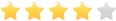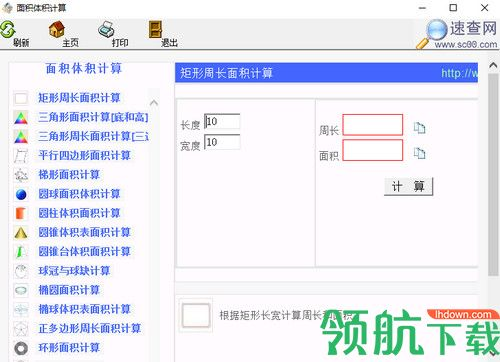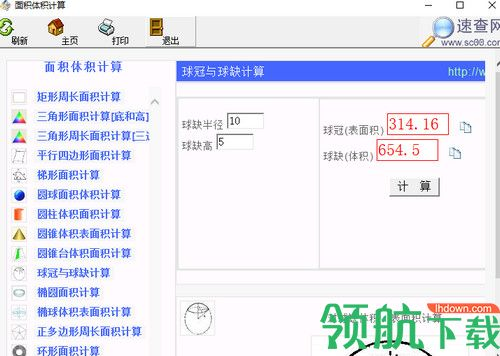• 软件大小：273.78KB
• 软件语言：中文
• 软件类型：国产软件
• 软件授权：免费版
• 更新时间：2019-09-30
• 软件类别：计算器类
• 软件官网：//www.lhdown.com/
• 应用平台：WinXP/Vista/Win7/Win8软件星级：41、矩形周长面积计算

2、三角形面积计算

3、平行四边形面积计算

4、圆球面积体积计算

5、球冠与球缺计算

6、椭圆面积计算

7、环形面积计算

### 面积体积计算公式大全

1、长方形的周长=(长+宽)×2 C=(a+b)×2

2、正方形的周长=边长×4 C=4a

3、长方形的面积=长×宽 S=ab

4、正方形的面积=边长×边长 S=a.a= a

5、三角形的面积=底×高÷2 S=ah÷2

6、平行四边形的面积=底×高 S=ah

7、梯形的面积=(上底+下底)×高÷2 S=(a+b)h÷2

8、直径=半径×2 d=2r 半径=直径÷2 r= d÷2

9、圆的周长=圆周率×直径=圆周率×半径×2 c=πd =2πr

10、圆的面积=圆周率×半径×半径 Ѕ=πr

11、长方体的表面积=(长×宽+长×高+宽×高)×2

12、长方体的体积 =长×宽×高 V =abh

13、正方体的表面积=棱长×棱长×6 S =6a

14、正方体的体积=棱长×棱长×棱长 V=a.a.a= a

15、圆柱的侧面积=底面圆的周长×高 S=ch

16、圆柱的表面积=上下底面面积+侧面积

S=2πr +2πrh=2π(d÷2) +2π(d÷2)h=2π(C÷2÷π) +Ch

17、圆柱的体积=底面积×高 V=Sh

V=πr h=π(d÷2) h=π(C÷2÷π) h

18、圆锥的体积=底面积×高÷3

V=Sh÷3=πr h÷3=π(d÷2) h÷3=π(C÷2÷π) h÷3

19、长方体(正方体、圆柱体)的体积=底面积×高 V=Sh

### 面积体积计算软件使用方法